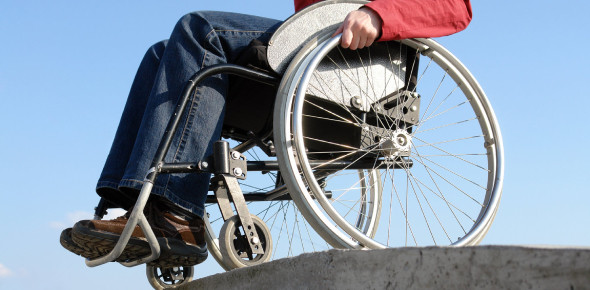# BP 344 Accessibility Law

100 Questions | Total Attempts: 6760SettingsSubject: BP 344 Answer the questions with the given choices. The entire questions are composed of basic and board exam type questions. Answer the questions sincerely with the time alloted. The main objective of the quiz is to let the examinees have the feel of answering questions while time pressured. Note: If you like to be notified for the updated questions and board exam events, add as on facebook: [email protected] Com If you have questions, feedback and suggestions regarding the quiz, email us at [email protected] Com. Alternatively, you can share your feedback at archiTALK corner for your profesional insights and questions.

• 1.
What is the least dimension of a parking slot for the disabled?
• A.

2.70 m

• B.

3.00 m

• C.

3.70 m

• D.

3.80 m

• 2.
What is the minimum dimension of a PWD toilet?
• A.

1.70 m

• B.

1.80 m

• C.

1.50 m

• D.

1.20 m

• 3.
What is the best way to provide for the convenience of both people in wheelchair and blind persons?
• A.

Railings

• B.

Tactile surface/ blocks in the immediate vicinity of crossings

• C.

Light controlled crossings with pedestrians phases and audible signals

• D.

Brightly painted zebra crossing

• 4.
What is the minimum width for a hallway?
• A.

1.10 m

• B.

1.20 m

• C.

1.40 m

• D.

1.50 m

• 5.
What is the distance of the Building Main Entrance to the Elevator?
• A.

60 m

• B.

20 m

• C.

30 m

• D.

40 m

• 6.
What is the minimum dimension of an elevator door width?
• A.

0.70 m

• B.

0.80 m

• C.

0.75 m

• D.

0.85 m

• 7.
What is the minimum size of an elevator car?
• A.

1.10 x 1.40 m

• B.

1.40 x 1.80 m

• C.

1.20 x 1.50 m

• D.

1.70 x 1.80 m

• 8.
What is the minimum size of an elevator shaft?
• A.

1.10 x 1.40 m

• B.

1.30 x 1.60 m

• C.

1.50 x 1.80 m

• D.

1.40 x 1.70 m

• 9.
Minimum door opening for elevators for a single door?
• A.

0.70 m

• B.

0.80 m

• C.

0.90 m

• D.

1.00 m

• 10.
What is the minimum size of Elevator Doors for the handicapped?
• A.

0.70 m

• B.

0.80 m

• C.

0.90 m

• D.

1.00 m

• 11.
What is the maximum distance of Elevators considering the handicapped?
• A.

30 m

• B.

45 m

• C.

60 m

• D.

43 M

• 12.
What is the minimum Doorway width under BP 344?
• A.

0.70 m

• B.

0.80 m

• C.

0.90 m

• D.

1.00 m

• 13.
Under BP 344. walks should have___  turn/ groove in order to guide the blind?
• A.

30 degrees

• B.

45 degrees

• C.

60 degrees

• D.

90 degrees

• 14.
Distance of parking for the disabled from building entrance?
• A.

30 m

• B.

50 m

• C.

60 m

• D.

80 m

• 15.
What is the minimum width for parking slots of PWD?
• A.

3.50 m

• B.

3.70 m

• C.

3.60 m

• D.

3.80 m

• 16.
For parking areas of PWD, a walkway between the front ends of parked cars shall have a minimum clear width of ____ ?
• A.

1.10 m

• B.

1.20 m

• C.

1.30 m

• D.

1.40 m

• 17.
Minimum entrance door opening?
• A.

0.70 m

• B.

0.80 m

• C.

0.90 m

• D.

1.00 m

• 18.
Minimum bedroom door opening?
• A.

0.70 m

• B.

0.80 m

• C.

0.90 m

• D.

1.00 m

• 19.
Minimum openings in a room ?
• A.

10% of wall area

• B.

12% of wall area

• C.

15% of wall are

• D.

20% of wall area

• 20.
Prefered height of Doorknobs?
• A.

0.82m

• B.

0.90m

• C.

1.00m

• D.

1.06 m

• 21.
A circle of ___ in diameter is a suitable guide in the planning of wheelchair turning spaces?
• A.

1.80 m

• B.

1.70 m

• C.

1.60 m

• D.

1.50 m

• 22.
Angle of turn of embossed tiles for the blind?
• A.

45 degrees

• B.

30 degrees

• C.

90 degrees

• D.

60 degrees

• 23.
Under the Accessibility Code, what is the maximum height of handrail?
• A.

0.70 m

• B.

0.75 m

• C.

0.80 m

• D.

0.90 m

• 24.
Min. clearance of a table from floor considering the handicapped?
• A.

45 mm

• B.

60 mm

• C.

70 mm

• D.

74 mm

• 25.
What is the preferred design for a stair considering users of crutches?
• A.

• B.

Open Riser Stairs

• C.

Slanted Nosing

• D.

Right Angle Stairs

Related TopicsBack to top